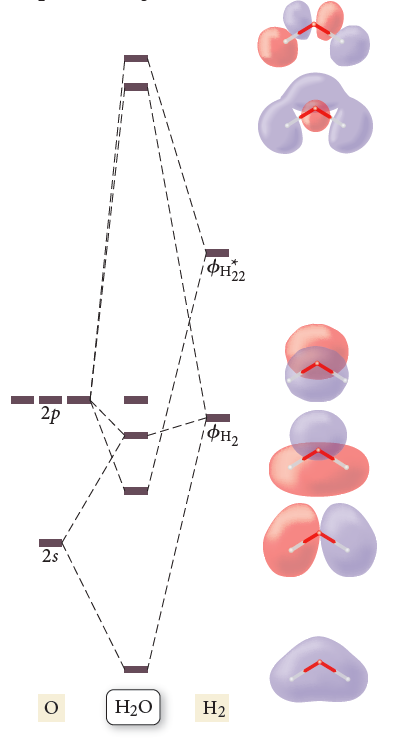# Problem: The results of a molecular orbital calculation for H2O are shown here. Examine each of the orbitals and classify them as bonding, antibonding, or nonbonding. Assign the correct number of electrons to the energy diagram. According to this energy diagram, is H2O stable? Explain.

###### FREE Expert Solution
91% (341 ratings)
###### Problem Details

The results of a molecular orbital calculation for H2O are shown here. Examine each of the orbitals and classify them as bonding, antibonding, or nonbonding. Assign the correct number of electrons to the energy diagram. According to this energy diagram, is H2O stable? Explain.